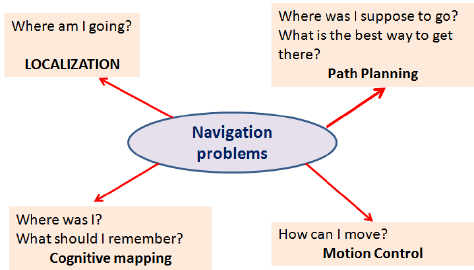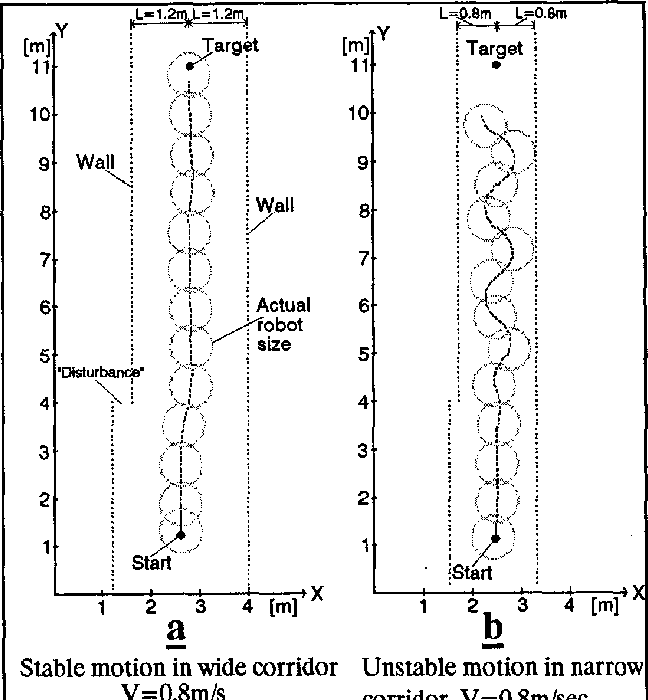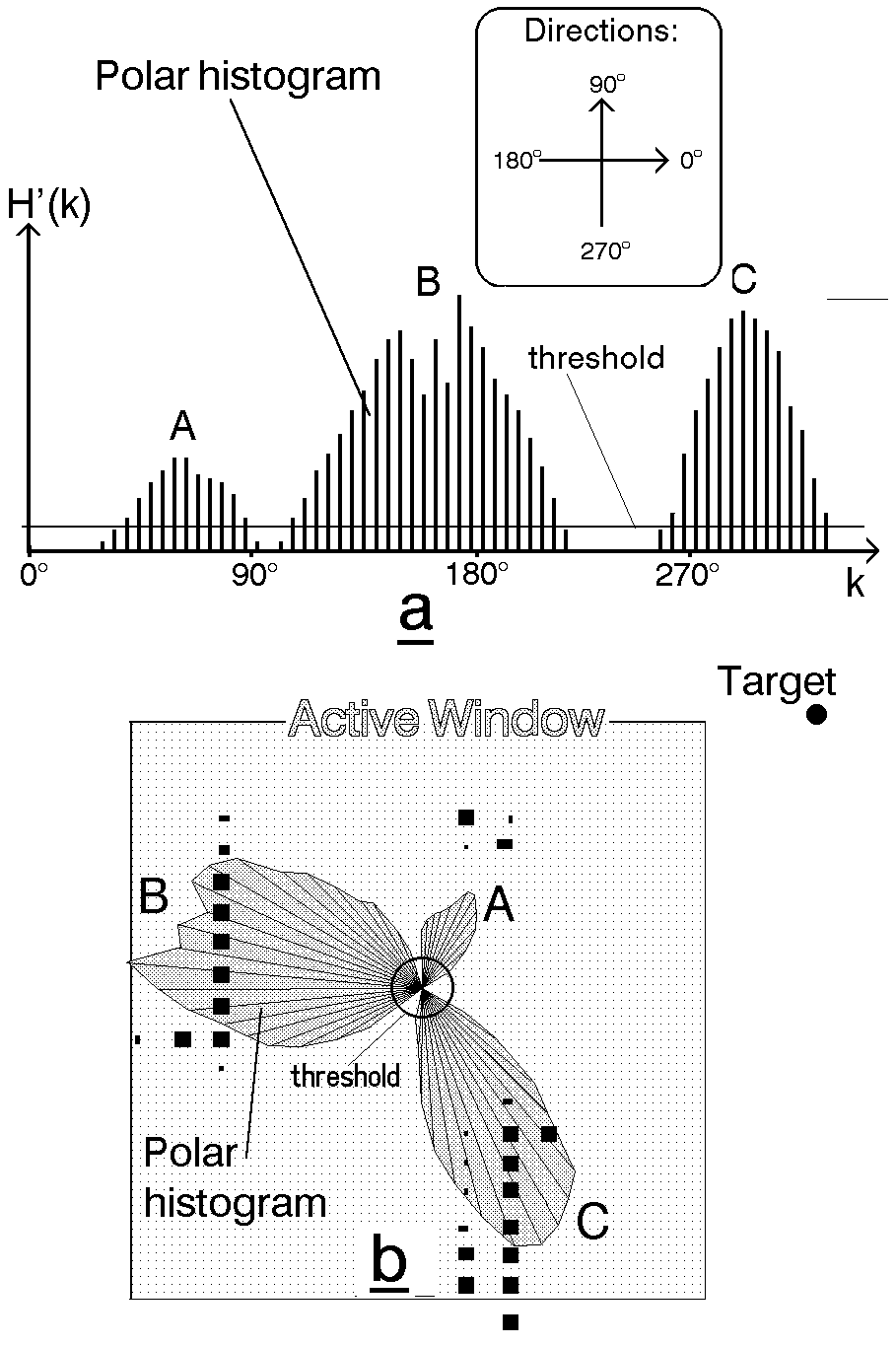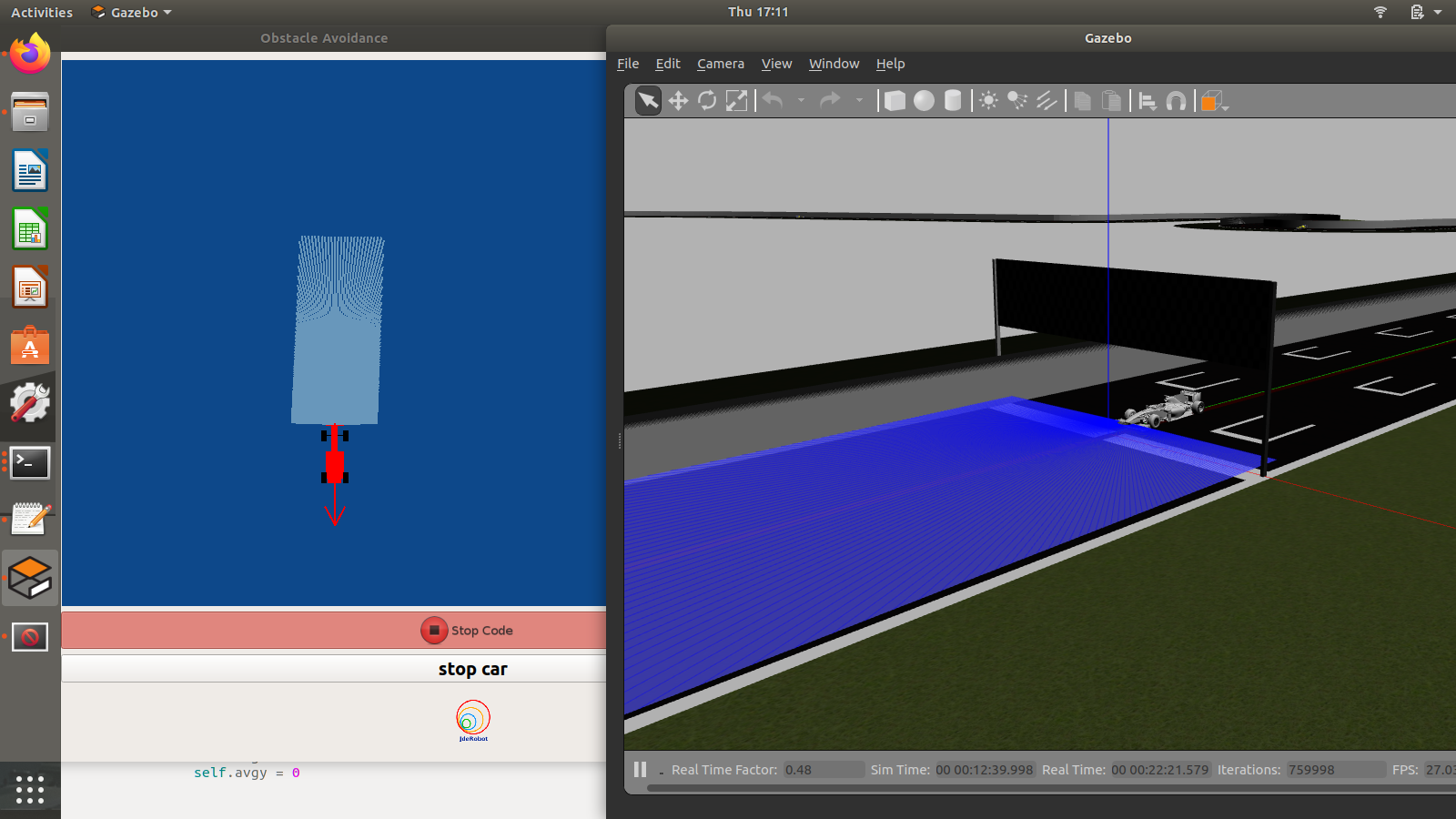## Objective

The objective of this practice is to implement the logic of the VFF navigation algorithm.

Navigation using VFF (Virtual Force Field), consists of:

• Each object in the environment generates a repulsive force towards the robot.

• Destiny generates an attractive force in the robot.

This makes it possible for the robot to go towards the target, distancing itself of the obstacles, so that their address is the vector sum of all the forces.

The solution can integrate one or more of the following levels of difficulty, as well as any other one that occurs to you:

• Go as quickly as possible

• Choose the safest way

• Obstacles in movement

• Robustness in situations of indecision (zero vector sum)

## Installation

Install the General Infrastructure of the JdeRobot Robotics Academy.

## How to run your solution?

Navigate to the obstacle_avoidance directory

cd exercises/obstacle_avoidance


Launch Gazebo with the f1_simple_circuitobstacles world through the command

roslaunch ./launch/obstacle_avoidance_f1.launch


Then you have to execute the academic application, which will incorporate your code:

python ./obstacle_avoidance_f1.py obstacle_avoidance_conf_f1.yml


## How to do the practice

To carry out the practice, you must edit the file MyAlgorithm.py and insert the control logic.

def execute(self):
self.currentTarget=self.getNextTarget()
self.targetx = self.currentTarget.getPose().x
self.targety = self.currentTarget.getPose().y

# TODO


### API

• pose3d.getPose3d().x - to get the position of the robot (x coordinate)
• pose3d.getPose3d().y - to obtain the position of the robot (y coordinate)
• pose3d.getPose3d().yaw - to get the orientation of the robot with regarding the map
• laser.getLaserData() - to obtain laser sensor data It is composed of 180 pairs of values: (0-180º distance in millimeters)
• motors.sendV() - to set and send the linear speed
• motors.sendW() - to set and send the angular velocity

### Own API

To simplify, the implementation of control points is offered. To use it, only two actions must be carried out:

1. Obtain the following point: self.currentTarget = self.getNextTarget()
2. Mark it as visited when necessary: self.currentTarget.setReached(True)

## Conversion of types

### Laser

    laser_data = self.laser.getLaserData ()

def parse_laser_data (laser_data):
laser = []
for i in range (laser_data.numLaser):
dist = laser_data.distanceData [i] /1000.0
laser + = [(dist, angle)]
return laser

laser_vectorized = []
for d, a in laser:
# (4.2.1) laser into GUI reference system
x = d * math.cos (a) * -1
y = d * math.sin (a) * -1
v = (x, y)
laser_vectorized + = [v]

laser_mean = np.mean (laser_vectorized, axis = 0)


### Coordinate system

def absolute2relative (x_abs, y_abs, robotx, roboty, robott):

# robotx, roboty are the absolute coordinates of the robot
# robott is its absolute orientation
# Convert to relatives
dx = x_abs - robotx
dy = y_abs - roboty

# Rotate with current angle
x_rel = dx * math.cos (-robott) - dy * math.sin (-robott)
y_rel = dx * math.sin (-robott) + dy * math.cos (-robott)

return x_rel, and y_rel


## Debugging

The graphical interface (GUI) allows to visualize each of the vectors of calculated forces. For this purpose, the following variables should be given value:

# Car direction
self.carx = 0.0
self.cary = 0.0

# Obstacles direction
self.obsx = 0.0
self.obsy = 0.0

# Average direction
self.avgx = 0.0
self.avgy = 0.0


As well as the destination that we have assigned:

# Current target
self.targetx = 0.0
self.targety = 0.0


## Theory

This exercise requires us to implement a local navigation algorithm called Virtual Force Field Algorithm. Following is the complete theory regarding this algorithm.

Robot Navigation involves all the related tasks and algorithms required to take a robot from point A to point B autonomously without making any collisions. It is a very well studied topic in Mobile Robotics, comprising volumes of books! The problem of Navigation is broken down into the following subproblems:

• Localisation: The robot needs to know where it is.
• Collision Avoidance: The robot needs to detect and avoid obstacles
• Mapping: The robot needs to remember its surroundings
• Planning: The robot needs to be able to plan a route to the point B
• Explore: The robot needs to be able to explore new terrainSome of the ways to achieve the task of Navigation are as follows:

• Vision Based: Computer Vision algorithms and optical sensors, like LIDAR sensors are used for Vision Based Navigation.

The problem of Path Planning in Navigation is dealt in 2 ways, which are Global Navigation and Local Navigation

Global Navigation involves the use of a map of the enviorment to plan a path from a point A to point B. The optimality of the path is decided based on the length of the path, the time taken to reach the target, using permanent roads etc. Global Positioning System(GPS) is one such example of Global Navigation. The algorithms used behind such systems may include Dijkstra, Best First or A* etc.

Once the global path is decided, it is broken down into suitable waypoints. The robot navigates through these waypoints in order to reach it’s destination. Local Navigation involves a dynamically changing path plan taking into consideration the changing surroundings and the vehicle constraints. Some examples of such algorithms would be Virtual Force Field, Follow Wall, Pledge Algorithm etc.

### Virtual Force Field Algorithm

The Virtual Force Field Algorithm works in the following steps:

• The robot assigns an attractive vector to the waypoint that points towards the waypoint.
• The robot assigns a repulsive vector to the obstacle according to its sensor readings that points away from the waypoint. This is done by summing all the vectors that are translated from the sensor readings.
• The robot follows the vector obtained by summing the target and obstacle vector.### Drawbacks

There are a few problems related to this algorithm:

• The robot tends to oscillate in narrow corridors, that is when the robot receives an obstacle vector simultaneously from opposite sides.
• The robot may not be able to enter narrow corridors in the first place!### Virtual Force Histogram Algorithm

This algorithm improves over the Virtual Force Field Algorithm, by using a data structure called the Polar Histogram. The robot maintains a histogram grid of the instantaneous sensor values received. Then, based on the threshold value set by the programmer, the program detects minimas(vallies) in the polar histogram. The angle corresponding to these values are then followed by the robot.Note: The exercise only requires us to implement Virtual Force Field Algorithm

## Hints

### Determining the Vectors

First of all, we need to generate the 3 required vectors, that are the Target Vector, Obstacle Vector and the Direction Vector.

### Target Vector

The target vector can be easily obtained by subtracting the position of the car from the position of the next waypoint.

In order to implement this on the GUI interface of the exercise, in addition to the vector obtained by subtracting, we need to apply a rotation to the vector as well. The purpose of rotation is to keep the target vector always in the direction of the waypoint and not in front of the car. You may try seeing this in your own implementation, or refer to the the illustrations

Refer to this webpage to know about the exact mathematical details of the implementation.

### Obstacle Vector

The obstacle vector is to be calculated from the sensor readings we obtain from the surroundings of the robot. Each obstacle in front of the car, is going to provide a repulsive vector, which we will add to obtain the resultant repulsive vector. Assign a repulsive vector, for each of the 180 sensor readings. The magnitude of the repulsive vector is inversly proportional to the magnitude of the sensor reading. Once, all the repulsive vectors are obtained they are all added, to get the resultant.

Note: There is a catch in this case, you may notice that most of the time in our implementation of the exercise we get an obstacle vector which is almost always pointing opposite to the direction in which we are supposed to head. This is a problem, as adding this vector directly to the target vector, would give a resultant which is more or less, quite not we expect. Hence, there is some trick we need to apply to solve this problem.Obstacle Vector without any obstacles in front of the car

### Direction Vector

Conventionally and according to the research paper of the VFF algorithm, in order to obtain the direction vector we should add the target vector and the obstacle vector. But, due to an inherent problem behind the calculation of the obstacle vector, we cannot simply add them to obtain the resultant.

A simple observation reveals that we are required to keep moving forward for the purpose of this exercise. Hence, the component of the direction vector in the direction of motion of the car, has no effect on the motion of the car. Therefore, we can simply leave it as a constant, while adjusting the vector responsible for the steering of the car. It is the steering, that will in fact, provide us with the obstacle avoidance capabilities. Hence, the steering is going to be controlled by the Direction Vector.

Also, please note that this is not the only solution to this problem. We may also add an offset vector in the direction of motion of the car to cancel the effect of the redundant component.